Скачать презентацию Price Discrimination A monopoly engages in price discrimination

4da9fd45e1d5492f48bbef6093d9a67d.ppt

• Количество слайдов: 15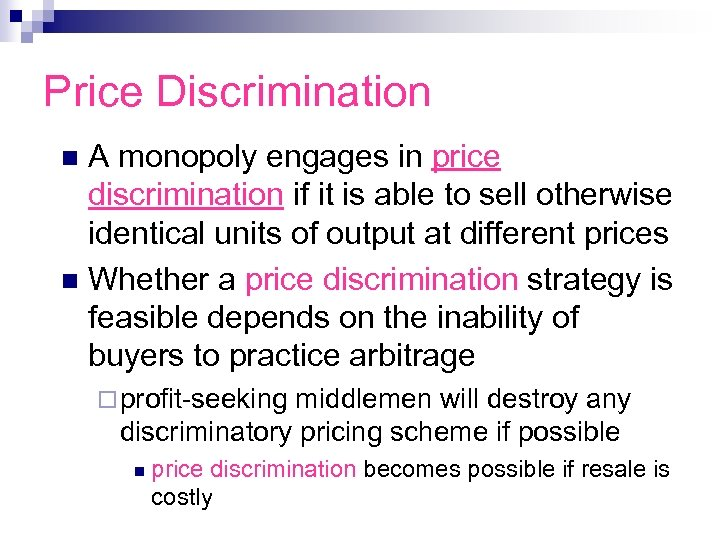Price Discrimination A monopoly engages in price discrimination if it is able to sell otherwise identical units of output at different prices n Whether a price discrimination strategy is feasible depends on the inability of buyers to practice arbitrage n ¨ profit-seeking middlemen will destroy any discriminatory pricing scheme if possible n price discrimination becomes possible if resale is costly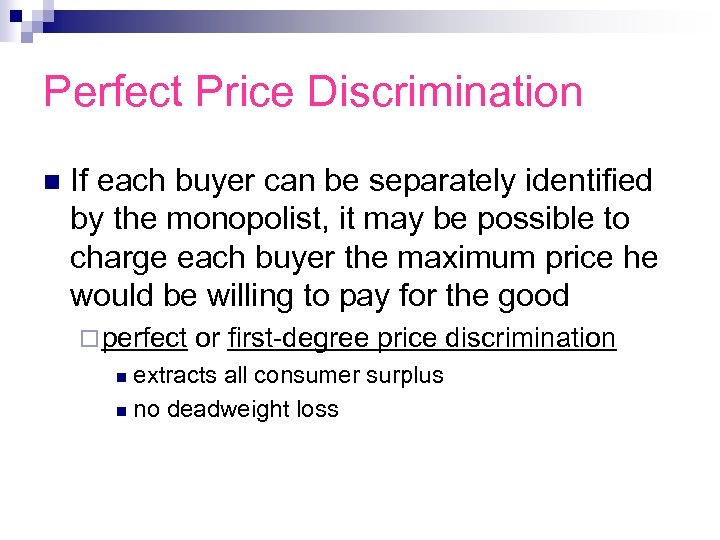Perfect Price Discrimination n If each buyer can be separately identified by the monopolist, it may be possible to charge each buyer the maximum price he would be willing to pay for the good ¨ perfect or first-degree price discrimination extracts all consumer surplus n no deadweight loss n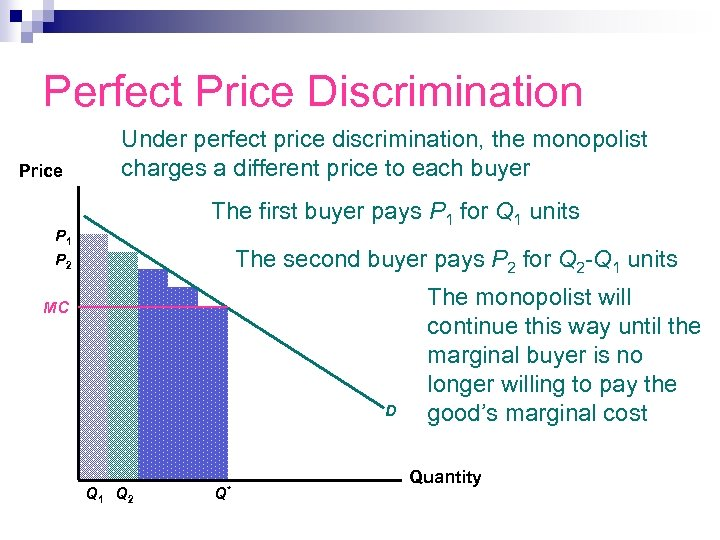Perfect Price Discrimination Price Under perfect price discrimination, the monopolist charges a different price to each buyer The first buyer pays P 1 for Q 1 units P 1 The second buyer pays P 2 for Q 2 -Q 1 units P 2 MC D Q 1 Q 2 Q* The monopolist will continue this way until the marginal buyer is no longer willing to pay the good’s marginal cost Quantity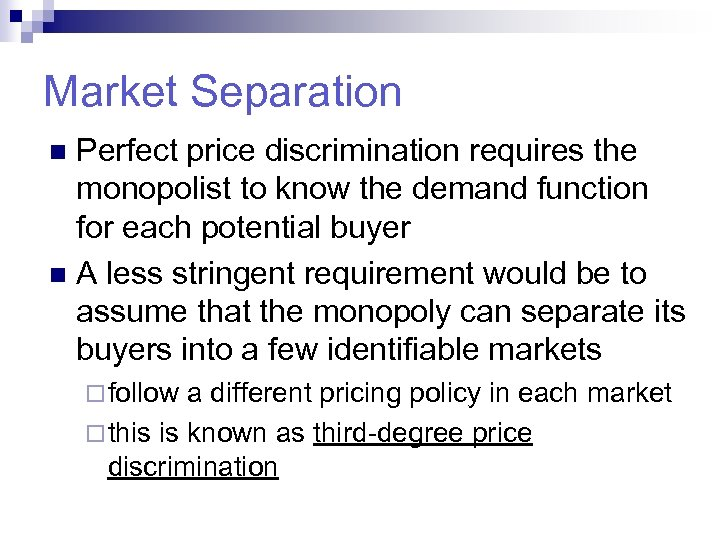Market Separation Perfect price discrimination requires the monopolist to know the demand function for each potential buyer n A less stringent requirement would be to assume that the monopoly can separate its buyers into a few identifiable markets n ¨ follow a different pricing policy in each market ¨ this is known as third-degree price discrimination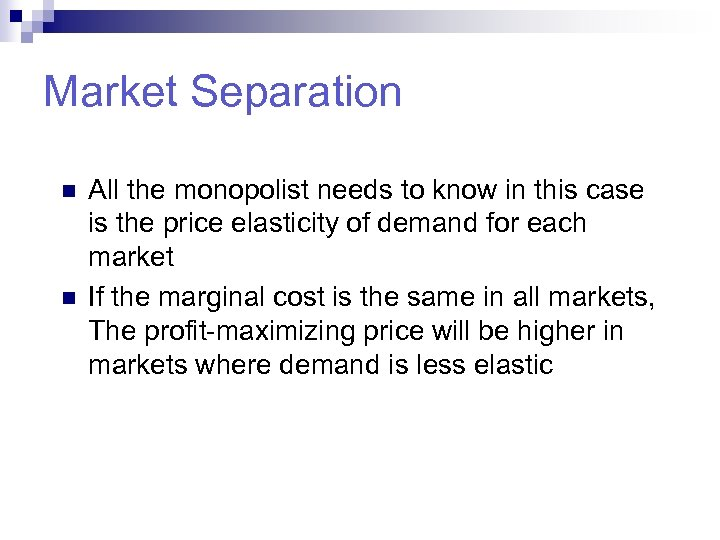Market Separation n n All the monopolist needs to know in this case is the price elasticity of demand for each market If the marginal cost is the same in all markets, The profit-maximizing price will be higher in markets where demand is less elastic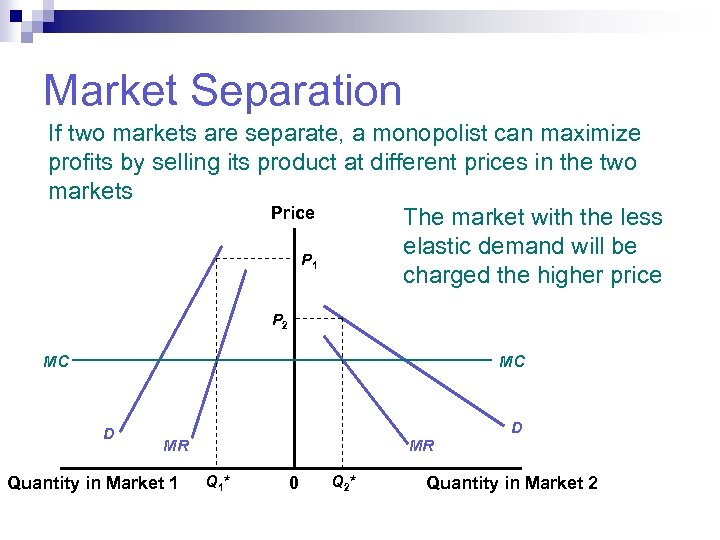Market Separation If two markets are separate, a monopolist can maximize profits by selling its product at different prices in the two markets Price The market with the less elastic demand will be P 1 charged the higher price P 2 MC MC D D MR Quantity in Market 1 MR Q 1* 0 Q 2* Quantity in Market 2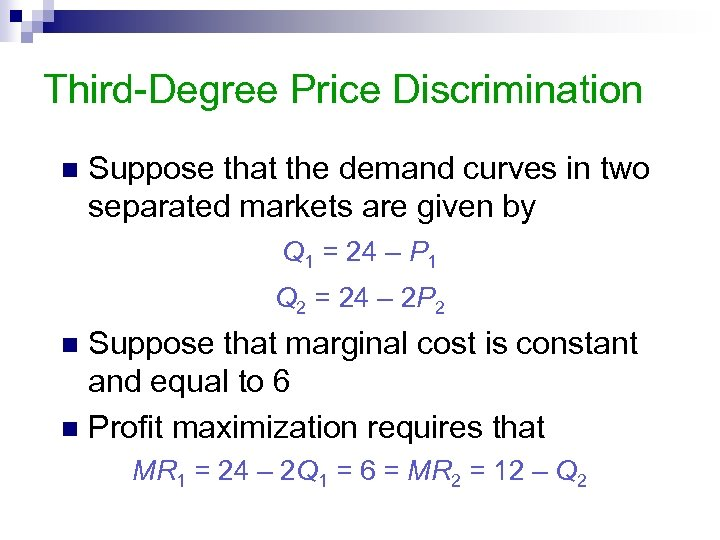Third-Degree Price Discrimination n Suppose that the demand curves in two separated markets are given by Q 1 = 24 – P 1 Q 2 = 24 – 2 P 2 Suppose that marginal cost is constant and equal to 6 n Profit maximization requires that n MR 1 = 24 – 2 Q 1 = 6 = MR 2 = 12 – Q 2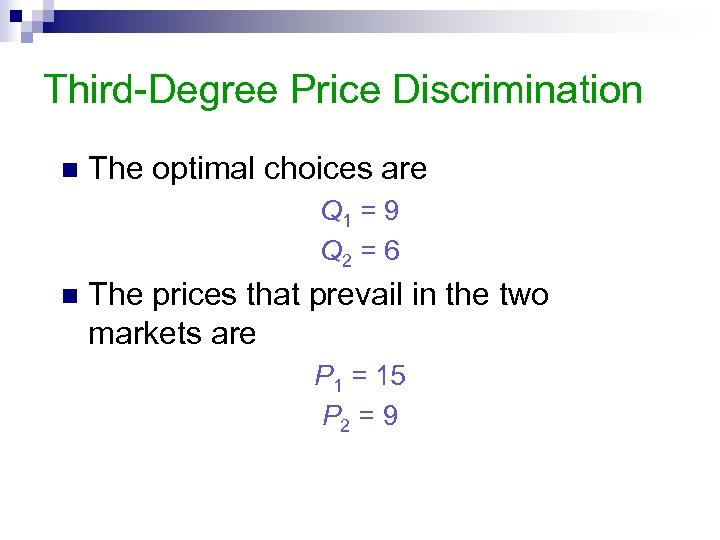Third-Degree Price Discrimination n The optimal choices are Q 1 = 9 Q 2 = 6 n The prices that prevail in the two markets are P 1 = 15 P 2 = 9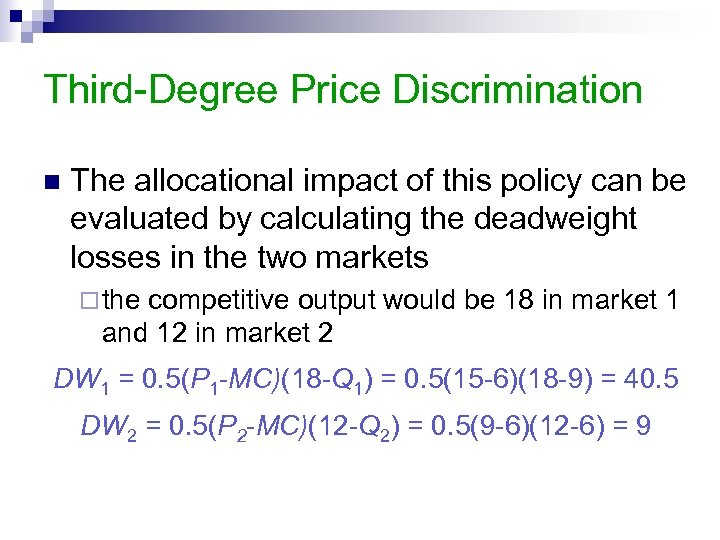Third-Degree Price Discrimination n The allocational impact of this policy can be evaluated by calculating the deadweight losses in the two markets ¨ the competitive output would be 18 in market 1 and 12 in market 2 DW 1 = 0. 5(P 1 -MC)(18 -Q 1) = 0. 5(15 -6)(18 -9) = 40. 5 DW 2 = 0. 5(P 2 -MC)(12 -Q 2) = 0. 5(9 -6)(12 -6) = 9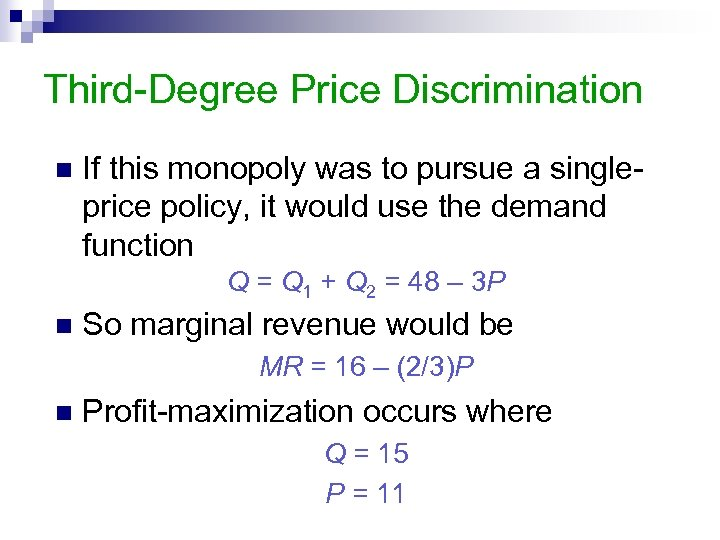Third-Degree Price Discrimination n If this monopoly was to pursue a singleprice policy, it would use the demand function Q = Q 1 + Q 2 = 48 – 3 P n So marginal revenue would be MR = 16 – (2/3)P n Profit-maximization occurs where Q = 15 P = 11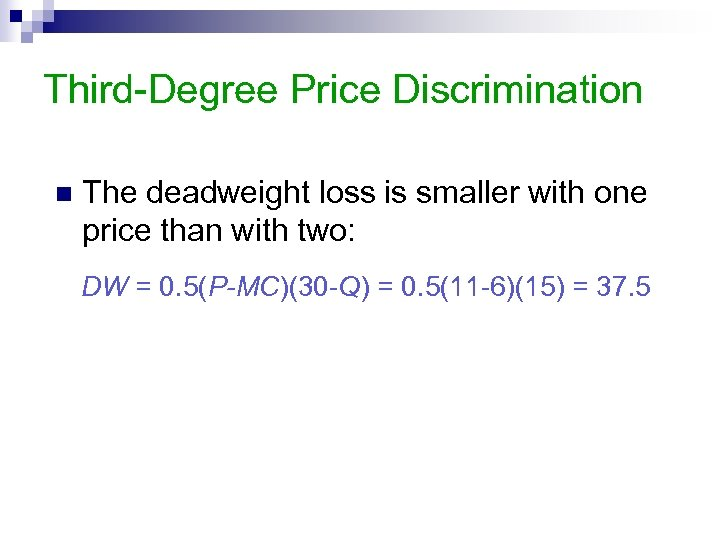Third-Degree Price Discrimination n The deadweight loss is smaller with one price than with two: DW = 0. 5(P-MC)(30 -Q) = 0. 5(11 -6)(15) = 37. 5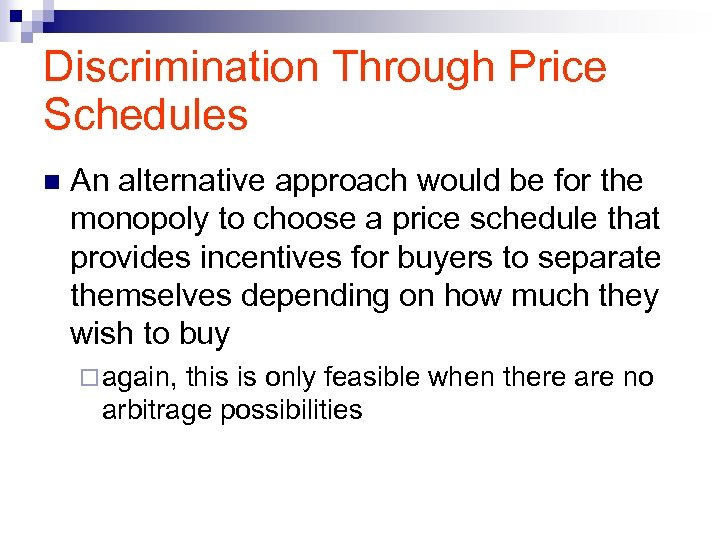Discrimination Through Price Schedules n An alternative approach would be for the monopoly to choose a price schedule that provides incentives for buyers to separate themselves depending on how much they wish to buy ¨ again, this is only feasible when there are no arbitrage possibilitiesTwo-Part Tariff n A linear two-part tariff occurs when buyers must pay a fixed fee for the right to consume a good and a uniform price for each unit consumed T(Q) = A + PQ n The monopolist’s goal is to choose A and P to maximize profits, given the demand for the product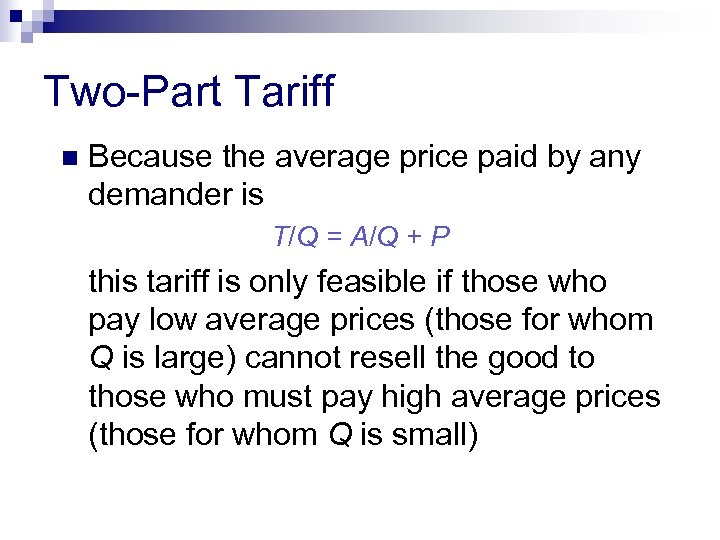Two-Part Tariff n Because the average price paid by any demander is T/Q = A/Q + P this tariff is only feasible if those who pay low average prices (those for whom Q is large) cannot resell the good to those who must pay high average prices (those for whom Q is small)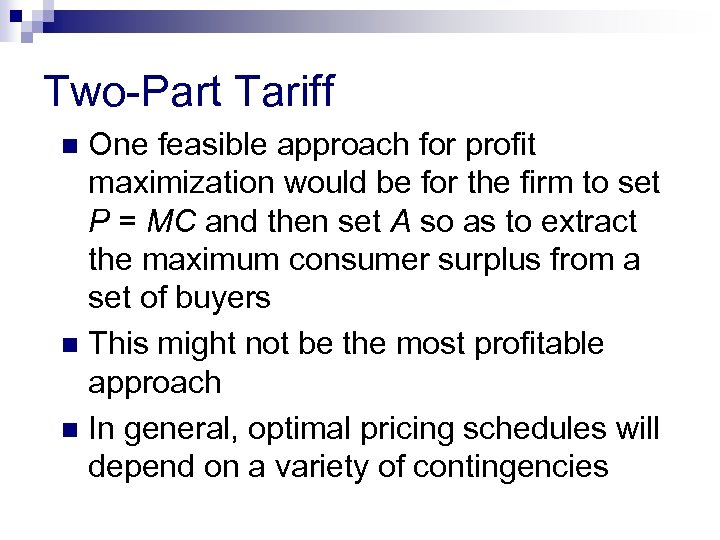Two-Part Tariff One feasible approach for profit maximization would be for the firm to set P = MC and then set A so as to extract the maximum consumer surplus from a set of buyers n This might not be the most profitable approach n In general, optimal pricing schedules will depend on a variety of contingencies n